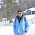### Coding Interview Questions Set 1

From this article, I will be sharing with you all a series of articles on coding interview questions.
So please stay connected for the latest set of questions.
It will be a good brainstorming exercise and will also prepare
you for coding interviews and will definitely boost your confidence.

So let's start,

1)Reverse of a string with only O(1) extra memory.

Solution:

var reverse = function(string) {
let result = ''
for(let i= string.length -1; i >= 0; i--){
result += string[i];
}
return result;
};

2)Fizz Buzz: Write a program that will accept a number n and will output number till n but for multiples of three it should output “Fizz” instead of the number and for the multiples of five output “Buzz”. For numbers which are multiples of both three and five output “FizzBuzz”.

Solution:

var fizzBuzz= function(n) {
const arr=[]
for(i=1; i<=n; i++){
if(i%15===0) arr.push("FizzBuzz")
else if(i%3===0) arr.push("Fizz")
else if(i%5===0) arr.push("Buzz")
else arr.push(i.toString())
}
return arr
};

3)Find a single element in an array of multiple elements where every element appears twice except the one you have to find. Do it in O(n) and with no extra memory.

Solutions:
There are multiple solutions that you can solve this in javascript,

a)

function findSingle(nums) {
return nums.reduce((prev, curr) => prev ^ curr, 0);
}

Logic:
The reduce method in JS reduces the array to a single value.
XOR will return 1 only on two different bits.
So if two numbers are the same, XOR will return 0.
Finally, only one number left.
A ^ A = 0 and A ^ B ^ A = B.

b)You have to find it out and have to post it in the comments section.
Hope to see someone in the comment section.

Please stay connected for coding interview questions set 2.

Don't forget to subscribe to this blog.

Written By:

Saurabh Joshi

1.const findSingle = (arr) =>
arr.reduce((acc, curr, idx) => {
if (acc.includes(curr)) {
acc.splice(acc.indexOf(curr), 1);
return acc;
}
else { return acc.concat([curr])}
}, [])

1.2.Your solution for (1) creates O(n) extra memory. For O(1), you need to reverse the input string in-place, with a fixed-size variable to facilitate 3-line-swaps:

var reverse = function(str) {

var tmp = '';
str = str.split('');

for(let i = 0; i < str.length / 2; i++) {
tmp = str[i];
str[i] = str[str.length - 1 - i];
str[str.length - 1 - i] = tmp;
}

return str.join('');
};

1.2.You allocate a new array in "result" instead of reversing in-place.

For input array length n, you allocate n more bytes to hold result.
For input length 2*n, allocate 2*n extra,
For input length 3*n, allocate 3*n extra.

Extra memory graph will look like f(x) = x

My method always allocates the same extra memory ("tmp"), no matter how input length increases. So the graph will look like f(x) = 1 or some constant. Flat horizontal graph.

Consider this similar problem, it also works in-place:
https://www.geeksforgeeks.org/reverse-individual-words-with-o1-extra-space/

3.Result is not an array it a string varia lble. And I am just appending it .

4.How much memory does your "result" allocate when input "string" is length 100? 200? 300?

3.Thanks now I got it

1.I am glad. Thank you for these posts. May your life be full of teachers.